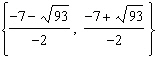Find the exact solution of the following quadratic equation by using the Quadratic Formula.

1. x2 – 7x = 60

2. -x2 + 7x + 11 = 0

Find the value of the discriminant. Then describe the number and type of roots for the equation.

3. -4x2 – 14x + 3 = 0

4. x2 – 3x + 8 = 0

5. What are the solutions of 5x2 – 16 = 0

Answers (1. {-5, 12}  2. see below  3. The discriminant is 244. Because the discriminant is greater than 0 and is not a perfect square, the roots are real and irrational.  4. The discriminant is –23. Because the discriminant is less than 0, the two roots are complex.  5. plus or minus 4 times the square root of 5 over 5.)(source)